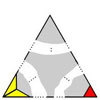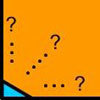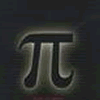# Resources tagged with: Rational and irrational numbers

Filter by: Content type:
Age range:
Challenge level:

There are 23 NRICH Mathematical resources connected to Rational and irrational numbers, you may find related items under The Number System and Place Value.

Broad Topics > The Number System and Place Value > Rational and irrational numbers### Repetitiously

##### Age 14 to 16Challenge Level

Can you express every recurring decimal as a fraction?### An Introduction to Irrational Numbers

##### Age 14 to 18

Tim Rowland introduces irrational numbers### Irrational Arithmagons

##### Age 16 to 18Challenge Level

Can you work out the irrational numbers that belong in the circles to make the multiplication arithmagon correct?### The Clue Is in the Question

##### Age 16 to 18Challenge Level

Starting with one of the mini-challenges, how many of the other mini-challenges will you invent for yourself?##### Age 16 to 18 ShortChallenge Level

Can you work out where the blue-and-red brick roads end?### Impossible Triangles?

##### Age 16 to 18Challenge Level

Which of these triangular jigsaws are impossible to finish?### The Square Hole

##### Age 14 to 16Challenge Level

If the yellow equilateral triangle is taken as the unit for area, what size is the hole ?### Equal Equilateral Triangles

##### Age 14 to 16Challenge Level

Can you make a regular hexagon from yellow triangles the same size as a regular hexagon made from green triangles ?### Impossible Square?

##### Age 16 to 18Challenge Level

Can you make a square from these triangles?### What Are Numbers?

##### Age 7 to 18

Ranging from kindergarten mathematics to the fringe of research this informal article paints the big picture of number in a non technical way suitable for primary teachers and older students.### Spirostars

##### Age 16 to 18Challenge Level

A spiropath is a sequence of connected line segments end to end taking different directions. The same spiropath is iterated. When does it cycle and when does it go on indefinitely?### An Introduction to Proof by Contradiction

##### Age 14 to 18

An introduction to proof by contradiction, a powerful method of mathematical proof.### The Dangerous Ratio

##### Age 11 to 14

This article for pupils and teachers looks at a number that even the great mathematician, Pythagoras, found terrifying.### All Is Number

##### Age 7 to 14### Rational Round

##### Age 16 to 18Challenge Level

Show that there are infinitely many rational points on the unit circle and no rational points on the circle x^2+y^2=3.### Proof Sorter - the Square Root of 2 Is Irrational

##### Age 16 to 18Challenge Level

Try this interactivity to familiarise yourself with the proof that the square root of 2 is irrational. Sort the steps of the proof into the correct order.### Approximations, Euclid's Algorithm & Continued Fractions

##### Age 16 to 18

This article sets some puzzles and describes how Euclid's algorithm and continued fractions are related.### Continued Fractions II

##### Age 16 to 18

In this article we show that every whole number can be written as a continued fraction of the form k/(1+k/(1+k/...)).### Making Rectangles, Making Squares

##### Age 11 to 14Challenge Level

How many differently shaped rectangles can you build using these equilateral and isosceles triangles? Can you make a square?### Rationals Between...

##### Age 14 to 16Challenge Level

What fractions can you find between the square roots of 65 and 67?### Rational Roots

##### Age 16 to 18Challenge Level

Given that a, b and c are natural numbers show that if sqrt a+sqrt b is rational then it is a natural number. Extend this to 3 variables.### Good Approximations

##### Age 16 to 18Challenge Level

Solve quadratic equations and use continued fractions to find rational approximations to irrational numbers.### Be Reasonable

##### Age 16 to 18Challenge Level

Prove that sqrt2, sqrt3 and sqrt5 cannot be terms of ANY arithmetic progression.